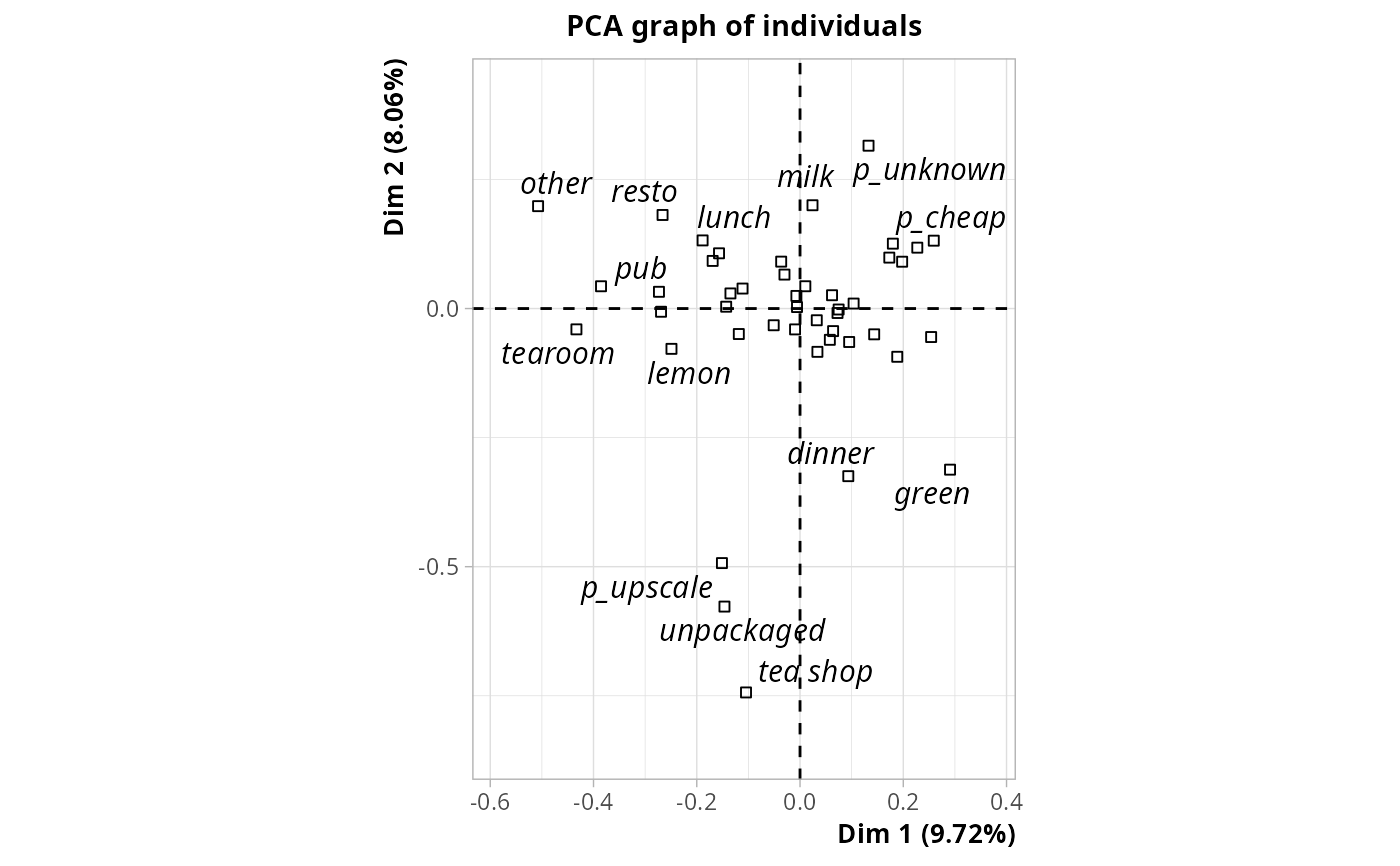Multiple Correspondence Analysis with Orthogonal Instrumental Variables

MCAoiv(X, Z, excl = NULL, row.w = NULL, ncp = 5)

## Arguments

X

data frame with only factors

Z

data frame of instrumental variables, which can be numeric or factors. It must have the same number of rows as X.

excl

numeric vector indicating the indexes of the "junk" categories (default is NULL). See getindexcat or use ijunk interactive function to identify these indexes. It may also be a character vector of junk categories, specified in the form "namevariable.namecategory" (for instance "gender.male").

row.w

Numeric vector of row weights. If NULL (default), a vector of 1 for uniform row weights is used.

ncp

number of dimensions kept in the results (by default 5)

## Details

Multiple Correspondence Analysis with Orthogonal Instrumental Variables consists in three steps : 1. Specific MCA of Y, keeping all the dimensions of the space 2. Computation of one linear regression for each dimension in the specific MCA, with individual coordinates as response and all variables in X as explanatory variables. 3. Principal Component Analysis of the set of residuals from the regressions in 2.

## Note

If there are NAs in Y, these NAs will be automatically considered as junk categories. If one desires more flexibility, Y should be recoded to add explicit factor levels for NAs and then excl option may be used to select the junk categories.

## Value

An object of class PCA from FactoMineR package, with X as supplementary variables, and an additional item :

ratio

the share of inertia not explained by the instrumental variables

.

## Author

Nicolas Robette

wcMCA, wcPCA, PCAoiv

## Examples

library(FactoMineR)
data(tea)
mcaoiv <- MCAoiv(tea[,1:18], tea[,19:22])
mcaoiv\$ratio
#>  0.9429018
plot(mcaoiv, choix = "ind", invisible = "ind", col.quali = "black")
#> Warning: ggrepel: 19 unlabeled data points (too many overlaps). Consider increasing max.overlaps